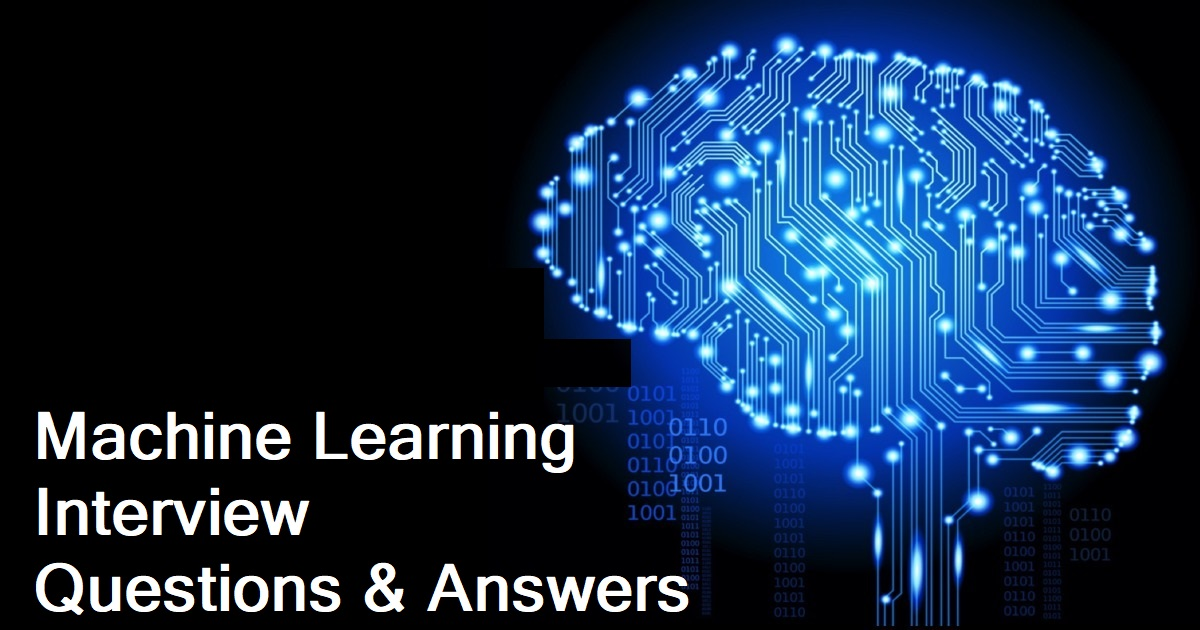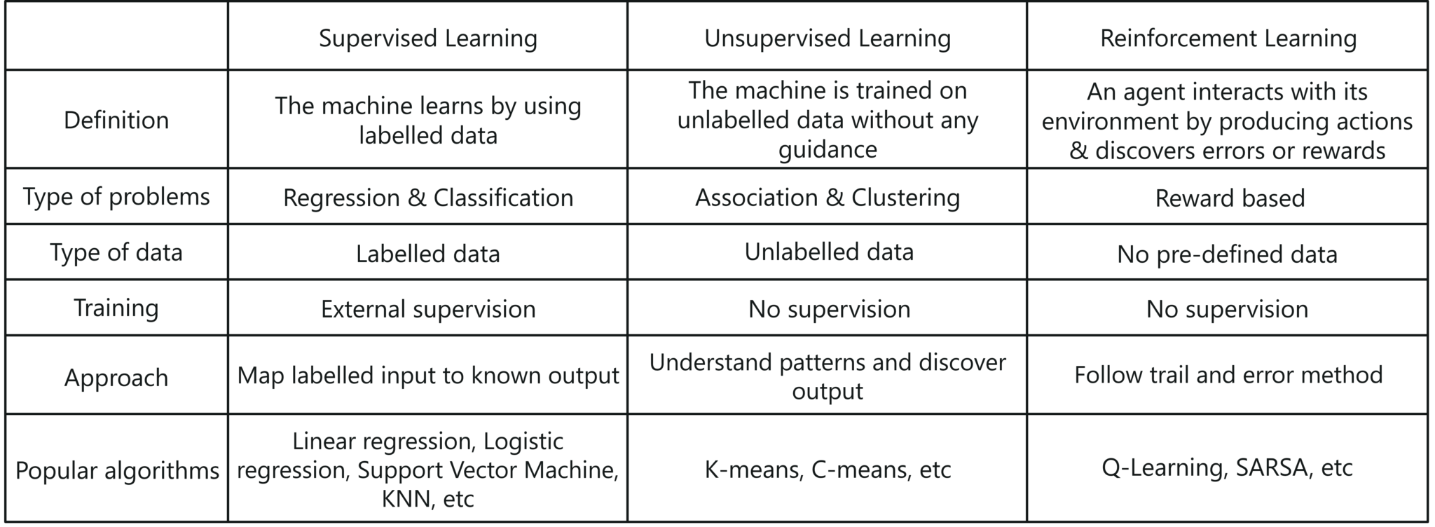Machine learning interview questions are an integral part of the data science interview and the path to becoming a data scientist, machine learning engineer, or data engineer. In order to help resolve that, here is a curated and created a list of key questions that you could see in a machine learning interview. There are some answers to go along with them so you don’t get stumped. You’ll be able to do well in any job interview (even for a machine learning internship) with after reading through this piece.1) What is Machine learning?

Machine learning is a branch of computer science which deals with system programming in order to automatically learn and improve with experience.  For example: Robots are programed so that they can perform the task based on data they gather from sensors. It automatically learns programs from data.

Also Read Part 2 for more Machine Learning Interview Questions

2) What are the different types of Machine Learning?There are three ways in which machines learn:

1. Supervised Learning
2. Unsupervised Learning
3. Reinforcement Learning

Supervised Learning:

Supervised learning is a method in which the machine learns using labeled data.

• It is like learning under the guidance of a teacher
• Training dataset is like a teacher which is used to train the machine
• Model is trained on a pre-defined dataset before it starts making decisions when given new data

Unsupervised Learning:

Unsupervised learning is a method in which the machine is trained on unlabelled data or without any guidance

• It is like learning without a teacher.
• Model learns through observation & finds structures in data.
• Model is given a dataset and is left to automatically find patterns and relationships in that dataset by creating clusters.

Reinforcement Learning:

Reinforcement learning involves an agent that interacts with its environment by producing actions & discovers errors or rewards.

• It is like being stuck in an isolated island, where you must explore the environment and learn how to live and adapt to the living conditions on your own.
• Model learns through the hit and trial method
• It learns on the basis of reward or penalty given for every action it performs

3) Mention the difference between Data Mining and Machine learning?

Machine learning relates with the study, design and development of the algorithms that give computers the capability to learn without being explicitly programmed.  While, data mining can be defined as the process in which the unstructured data tries to extract knowledge or unknown interesting patterns.  During this process machine, learning algorithms are used.

4) Explain Classification and Regression?5) What is ‘Overfitting’ in Machine learning?

In machine learning, when a statistical model describes random error or noise instead of underlying relationship ‘overfitting’ occurs.  When a model is excessively complex, overfitting is normally observed, because of having too many parameters with respect to the number of training data types. The model exhibits poor performance which has been overfit.

6) Why overfitting happens?

The possibility of overfitting exists as the criteria used for training the model is not the same as the criteria used to judge the efficacy of a model.

7) How can you avoid overfitting?

By using a lot of data overfitting can be avoided, overfitting happens relatively as you have a small dataset, and you try to learn from it. But if you have a small database and you are forced to come with a model based on that. In such situation, you can use a technique known as cross validation. In this method the dataset splits into two section, testing and training datasets, the testing dataset will only test the model while, in training dataset, the datapoints will come up with the model.

In this technique, a model is usually given a dataset of a known data on which training (training data set) is run and a dataset of unknown data against which the model is tested. The idea of cross validation is to define a dataset to “test” the model in the training phase.

8) What is inductive machine learning?

The inductive machine learning involves the process of learning by examples, where a system, from a set of observed instances tries to induce a general rule.

9) What are the five popular algorithms of Machine Learning?

• Decision Trees
• Neural Networks (back propagation)
• Probabilistic networks
• Nearest Neighbour
• Support vector machines

10) What are the three stages to build the hypotheses or model in machine learning?

• Model building
• Model testing
• Applying the model

11) What is the standard approach to supervised learning?

The standard approach to supervised learning is to split the set of example into the training set and the test.

12) What is ‘Training set’ and ‘Test set’?

In various areas of information science like machine learning, a set of data is used to discover the potentially predictive relationship known as ‘Training Set’. Training set is an examples given to the learner, while Test set is used to test the accuracy of the hypotheses generated by the learner, and it is the set of example held back from the learner. Training set are distinct from Test set.

13) List down various approaches for machine learning?

The different approaches in Machine Learning are

• Concept Vs. Classification Learning
• Symbolic Vs. Statistical Learning
• Inductive Vs. Analytical Learning

14) What is not Machine Learning?

• Artificial Intelligence
• Rule based inference

15) Explain what is the function of ‘Unsupervised Learning’?

• Find clusters of the data
• Find low-dimensional representations of the data
• Find interesting directions in data
• Interesting coordinates and correlations
• Find novel observations/ database cleaning

16) Explain what is the function of ‘Supervised Learning’?

• Classifications
• Speech recognition
• Regression
• Predict time series
• Annotate strings

17) What is algorithm independent machine learning?

Machine learning in where mathematical foundations is independent of any particular classifier or learning algorithm is referred as algorithm independent machine learning?

18) What is the difference between artificial learning and machine learning?

Designing and developing algorithms according to the behaviours based on empirical data are known as Machine Learning.  While artificial intelligence in addition to machine learning, it also covers other aspects like knowledge representation, natural language processing, planning, robotics etc.

19) What is classifier in machine learning?

A classifier in a Machine Learning is a system that inputs a vector of discrete or continuous feature values and outputs a single discrete value, the class.

20) What are the advantages of Naive Bayes?

In Naïve Bayes classifier will converge quicker than discriminative models like logistic regression, so you need less training data.  The main advantage is that it can’t learn interactions between features.

Also Read Part 2 for more Machine Learning Interview Questions

21) In what areas Pattern Recognition is used?

Pattern Recognition can be used in

• Computer Vision
• Speech Recognition
• Data Mining
• Statistics
• Informal Retrieval
• Bio-Informatics

22) What is Genetic Programming?

Genetic programming is one of the two techniques used in machine learning. The model is based on the testing and selecting the best choice among a set of results.

23) What is Inductive Logic Programming in Machine Learning?

Inductive Logic Programming (ILP) is a subfield of machine learning which uses logical programming representing background knowledge and examples.

24) What is Model Selection in Machine Learning?

The process of selecting models among different mathematical models, which are used to describe the same data set is known as Model Selection. Model selection is applied to the fields of statistics, machine learning and data mining.

25) What are the two methods used for the calibration in Supervised Learning?

The two methods used for predicting good probabilities in Supervised Learning are

• Platt Calibration
• Isotonic Regression

These methods are designed for binary classification, and it is not trivial.

26) Which method is frequently used to prevent overfitting?

When there is sufficient data ‘Isotonic Regression’ is used to prevent an overfitting issue.

27) What is Perceptron in Machine Learning?

In Machine Learning, Perceptron is an algorithm for supervised classification of the input into one of several possible non-binary outputs.

28) Explain the two components of Bayesian logic program?

Bayesian logic program consists of two components.  The first component is a logical one; it consists of a set of Bayesian Clauses, which captures the qualitative structure of the domain.  The second component is a quantitative one, it encodes the quantitative information about the domain.

29) What are Bayesian Networks (BN)?

Bayesian Network is used to represent the graphical model for probability relationship among a set of variables.

30) Why instance based learning algorithm sometimes referred as Lazy learning algorithm?

Instance based learning algorithm is also referred as Lazy learning algorithm as they delay the induction or generalization process until classification is performed.

31) What are the two classification methods that SVM (Support Vector Machine) can handle?

• Combining binary classifiers
• Modifying binary to incorporate multiclass learning

32) What is ensemble learning?

To solve a particular computational program, multiple models such as classifiers or experts are strategically generated and combined. This process is known as ensemble learning.

33) Why ensemble learning is used?

Ensemble learning is used to improve the classification, prediction,function approximation etc. of a model.

34) When to use ensemble learning?

Ensemble learning is used when you build component classifiers that are more accurate and independent from each other.

35) What are the two paradigms of ensemble methods?

The two paradigms of ensemble methods are

• Sequential ensemble methods
• Parallel ensemble methods

Also Read Part 2 for more Machine Learning Interview Questions

1.Riya says: